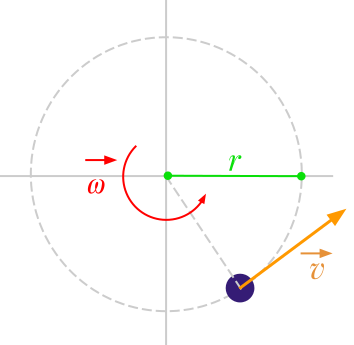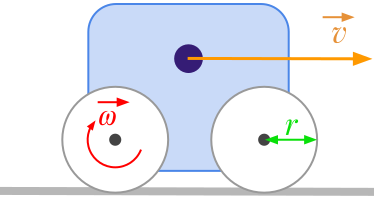Question #280

# How to convert linear velocity to angular velocity?The formula to convert linear speed ﻿﻿ expressed in meters per second [﻿﻿] to angular velocity ﻿﻿ expressed in radians per second [﻿﻿] is given by:

﻿﻿

where:

• ﻿﻿ is the linear velocity expressed in meters per second (﻿﻿)
• ﻿﻿ is the angular velocity expressed in radians per second (﻿﻿)
• ﻿﻿ is the radius expressed in meters (﻿﻿)For example, let's considere a vehicle with wheels having a radius of 10 centimetres. If the vehicle is moving at the linear velocity of 2 m/s, the rotation speed of the wheels is given by:

﻿﻿

You'll find on this page an online converter from linear to angular velocity.

22 events in history

Icons proudly provided by Friconix.